﻿ 高斯判别模型对人类心脏疾病的预测分析

高斯判别模型对人类心脏疾病的预测分析Predictive Analysis of Gauss Discriminant Model for Human Heart Disease

Abstract: With the spread of modern lifestyles and the use of personal computers for office work, sitting for long periods of time reduces the need for physical exercise and can lead to heart disease. In this paper, based on personal body information, classification prediction model is used to establish an early warning mechanism for individual heart disease. Descriptive statistical analysis was performed on age, sex, type of chest pain, resting blood pressure and continuous values such as cholesterol content, resting blood pressure and maximum heart rate. Discriminant learning algorithms, such as logistic regression, provide a new way. From the perspective of Bayesian prior, a rigorous Gaussian discriminant model is introduced in the generation model.

1. 引言

2. 对心脏疾病的描述性统计分析。

2.1. 对样本数据中主要变量的介绍Table 1. Independent variable name explanation

2.2. 离散型数据的描述性统计分析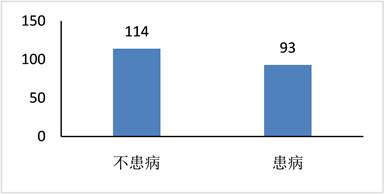Figure 1. Bar chart of whether women are sick or not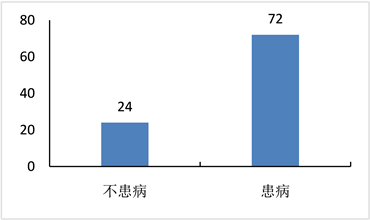Figure 2. Bar chart of whether men are sick or not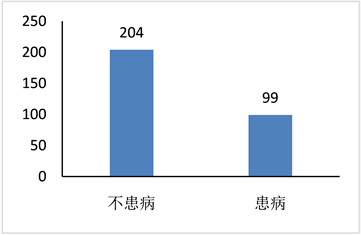Figure 3. Bar chart of patients with angina pectoris

2.3. 连续型数据的描述性统计分析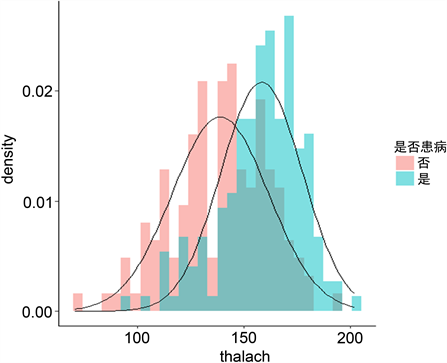Figure 4. Maximum heart rate and disease histogramFigure 5. The correlation between continuous variables

3. 高斯判别分析

3.1. 模型评价指标介绍

$P\left(y|x\right)=\frac{P\left(x|y\right)P\left(y\right)}{P\left(x\right)}$ (1)

$P\left(x\right)=P\left(x|{y}_{1}\right)P\left({y}_{1}\right)+P\left(x|{y}_{2}\right)\left({y}_{2}\right)$ (2)

$\text{PPV}=\frac{\text{TP}}{\text{TP}+\text{FP}}$ (3)

$\text{TPR}=\frac{\text{TP}}{\text{TP}+\text{FN}}$ (4)

$\text{F}1=\frac{2\text{TP}}{2\text{TP}+\text{FN}+\text{FP}}$ (5)

3.2. 模型结果分析Figure 6. Experimental results of the training set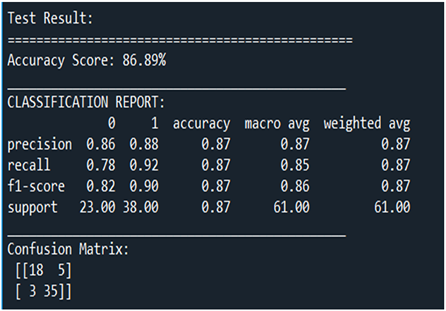Figure 7. Experimental results of the testing set

4. 结论

 王学民. 应用多元分析[M]. 上海: 上海财经大学出版社, 2004.

 佚名. 统计学习方法[M]. 北京: 清华大学出版社, 2012.

 吴喜之. 复杂数据统计方法[M]. 北京: 中国人民大学出版社, 2013.

Top